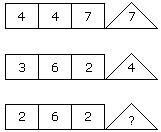# Verbal Reasoning - Character Puzzles - Discussion

### Discussion :: Character Puzzles - Character Puzzles 1 (Q.No.40)

40.

Which one will replace the question mark ?[A]. 2 [B]. 4 [C]. 6 [D]. 8

Explanation:

(4 x 7) % 4 = 7

and (6 x 2) % 3 = 4

Therefore, (6 x 2) % 2 = 6.

 Zapper said: (Jan 13, 2014) 4*1 = 4 So 1*7 = 7. 3*2 = 6 So 2*2 = 4. Like above, 2*3 = 6 so 3*2 = 6.

 Arm said: (Oct 7, 2016) Right @Zapper.

 Rounak said: (Jun 8, 2018) 4/4 * 7=7 in the same way 6/2*3=6.